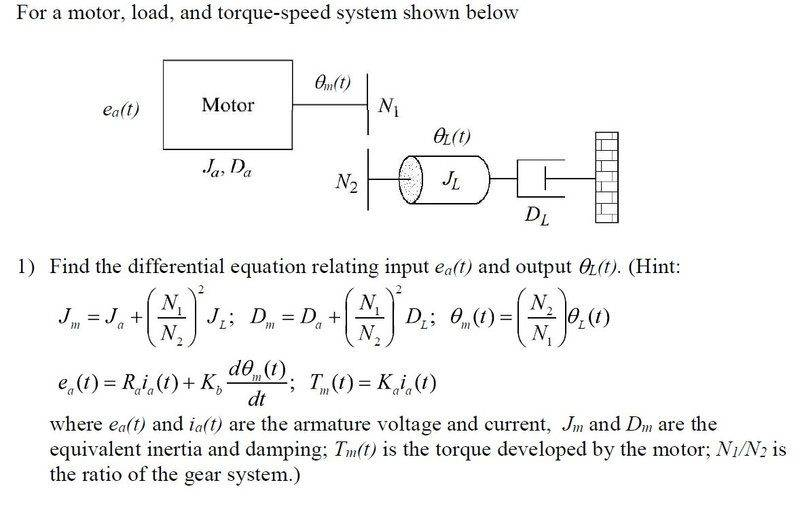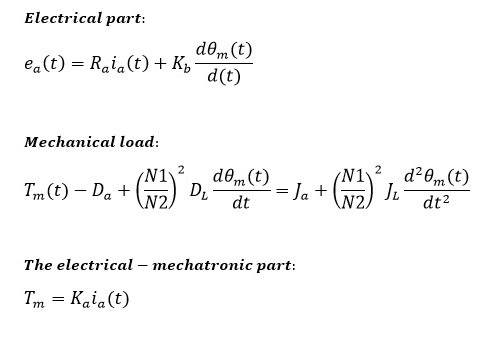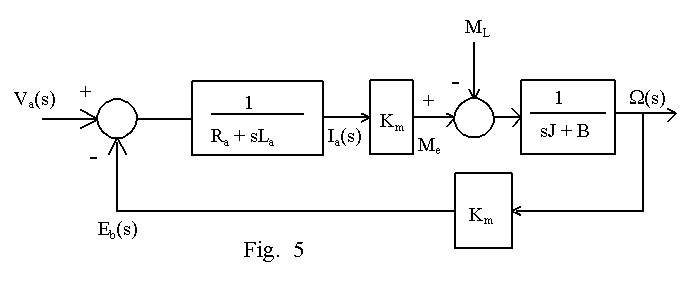# DC motor differential equation

• Engineering
Scott77
User has been reminded to use the Homework Help Template in schoolwork thread startsThis question involves finding the transfer function for the system, but I first need to get the differential equations correct. Have I set up the gearbox correctly?## Answers and Replies

Gold Member
This question involves finding the transfer function for the system, but I first need to get the differential equations correct.

Well, the transfer function is a differential equation. Using Laplace transform it may be written:

H(s) = output(s)/input(s)

When expressing the transfer function by its Laplace transform, it becomes much easier to calculate at controller.

I have some questions:

- Is it a DC-motor ?
- What is the input: Current or voltage?
- What is the preferred output: Speed or position?
- Is the armature damped ( Da )?
- What about the self induction in the armature, La?

If it's a DC-motor, you should come up with something like this, as for the transfer function:Use Masons rule to determine the transfer function as for the motor: ω(s) / Va(s)

Scott77
This is a system modeling question. That diagram and the hints is all the info we got, but we were told to ignore the armature inductance. I don't think it matters what the input type and output type are for this type of question...

Gold Member
Of course the input and output type matters. If you change the type of input or output, the motors behaviour will change.

In the model, choosing ω(s) as output, the transfer function will be H(s). Choosing θ(s) as output, the transfer function will be H(s)/s:
θ(s) = ω(s)/s.

Scott77
The input is ea(t) and the output is θL(t) like the question states, that is all I know.

Gold Member
Ok, then use these types as input/output.

Setting La = 0, you will get a 2. order transfer function.

Scott77
So is does this look correct?Gold Member
Ka and Kb have the same value, but the units are [Nm/A] and [Vs] respectively.

There are people that can prove it.